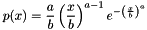#Boost C++ Libraries

...one of the most highly regarded and expertly designed C++ library projects in the world.

This is the documentation for an old version of Boost. Click here to view this page for the latest version.

## Class template weibull_distribution

boost::random::weibull_distribution

## Synopsis

```// In header: <boost/random/weibull_distribution.hpp>

template<typename RealType = double>
class weibull_distribution {
public:
// types
typedef RealType result_type;
typedef RealType input_type;

// member classes/structs/unions

class param_type {
public:
// types
typedef weibull_distribution distribution_type;

// construct/copy/destruct
explicit param_type(RealType = 1.0, RealType = 1.0);

// public member functions
RealType a() const;
RealType b() const;

// friend functions
template<typename CharT, typename Traits>
friend std::basic_ostream< CharT, Traits > &
operator<<(std::basic_ostream< CharT, Traits > &, const param_type &);
template<typename CharT, typename Traits>
friend std::basic_istream< CharT, Traits > &
operator>>(std::basic_istream< CharT, Traits > &, const param_type &);
friend bool operator==(const param_type &, const param_type &);
friend bool operator!=(const param_type &, const param_type &);
};

// construct/copy/destruct
explicit weibull_distribution(RealType = 1.0, RealType = 1.0);
explicit weibull_distribution(const param_type &);

// public member functions
template<typename URNG> RealType operator()(URNG &) const;
template<typename URNG>
RealType operator()(URNG &, const param_type &) const;
RealType a() const;
RealType b() const;
RealType min() const;
RealType max() const;
param_type param() const;
void param(const param_type &);
void reset();

// friend functions
template<typename CharT, typename Traits>
friend std::basic_ostream< CharT, Traits > &
operator<<(std::basic_ostream< CharT, Traits > &,
const weibull_distribution &);
template<typename CharT, typename Traits>
friend std::basic_istream< CharT, Traits > &
operator>>(std::basic_istream< CharT, Traits > &,
const weibull_distribution &);
friend bool operator==(const weibull_distribution &,
const weibull_distribution &);
friend bool operator!=(const weibull_distribution &,
const weibull_distribution &);
};```

## Description

The Weibull distribution is a real valued distribution with two parameters a and b, producing values >= 0.

It has.

### `weibull_distribution` public construct/copy/destruct

1. `explicit weibull_distribution(RealType a = 1.0, RealType b = 1.0);`

Constructs a `weibull_distribution` from its "a" and "b" parameters.

Requires: a > 0 && b > 0

2. `explicit weibull_distribution(const param_type & param);`

Constructs a `weibull_distribution` from its parameters.

### `weibull_distribution` public member functions

1. `template<typename URNG> RealType operator()(URNG & urng) const;`

Returns a random variate distributed according to the `weibull_distribution`.

2. ```template<typename URNG>
RealType operator()(URNG & urng, const param_type & param) const;```

Returns a random variate distributed accordint to the Weibull distribution with parameters specified by `param`.

3. `RealType a() const;`

Returns the "a" parameter of the distribution.

4. `RealType b() const;`

Returns the "b" parameter of the distribution.

5. `RealType min() const;`

Returns the smallest value that the distribution can produce.

6. `RealType max() const;`

Returns the largest value that the distribution can produce.

7. `param_type param() const;`

Returns the parameters of the distribution.

8. `void param(const param_type & param);`

Sets the parameters of the distribution.

9. `void reset();`

Effects: Subsequent uses of the distribution do not depend on values produced by any engine prior to invoking reset.

### `weibull_distribution` friend functions

1. ```template<typename CharT, typename Traits>
friend std::basic_ostream< CharT, Traits > &
operator<<(std::basic_ostream< CharT, Traits > & os,
const weibull_distribution & wd);```

Writes a `weibull_distribution` to a `std::ostream`.

2. ```template<typename CharT, typename Traits>
friend std::basic_istream< CharT, Traits > &
operator>>(std::basic_istream< CharT, Traits > & is,
const weibull_distribution & wd);```

Reads a `weibull_distribution` from a `std::istream`.

3. ```friend bool operator==(const weibull_distribution & lhs,
const weibull_distribution & rhs);```

Returns true if the two instances of `weibull_distribution` will return identical sequences of values given equal generators.

4. ```friend bool operator!=(const weibull_distribution & lhs,
const weibull_distribution & rhs);```

Returns true if the two instances of `weibull_distribution` will return different sequences of values given equal generators.

 Copyright © 2000-2005 Jens MaurerCopyright © 2009, 2010 Steven Watanabe Distributed under the Boost Software License, Version 1.0. (See accompanying file LICENSE_1_0.txt or copy at http://www.boost.org/LICENSE_1_0.txt)